# SIMPLIS - The 5 Minute Story

SIMPLIS (SIMulation of Piecewise LInear Systems) is a circuit simulator specifically designed to handle the simulation challenges of switching power systems. Like SPICE, SIMPLIS works at the component level but typically can perform a transient analysis of a switching circuit 10 to 50 times faster. For switching power systems, the piecewise linear (PWL) modeling and simulation techniques employed by SIMPLIS result in qualitatively superior convergence behavior compared to SPICE.

• Run the simulation with the default analyses. You can launch a simulation by either:
• clicking on the schematic Run Schematic icon,• or pressing the F9 function key,
• or clicking on the Simulator | Run Schematic menu item.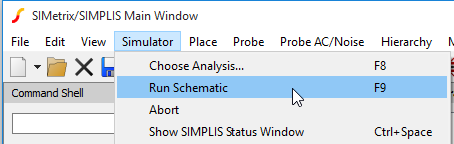• This SIMPLIS schematic is set up to find the steady-state Periodic Operating Point (POP) of the circuit, do an AC analysis and then do a step-load transient. Each of these three analyses takes about 1 or 2 seconds. The graph viewer opens with the following results.
• On the center tab, we see the time domain simulation of the system response to a pulse load on the output.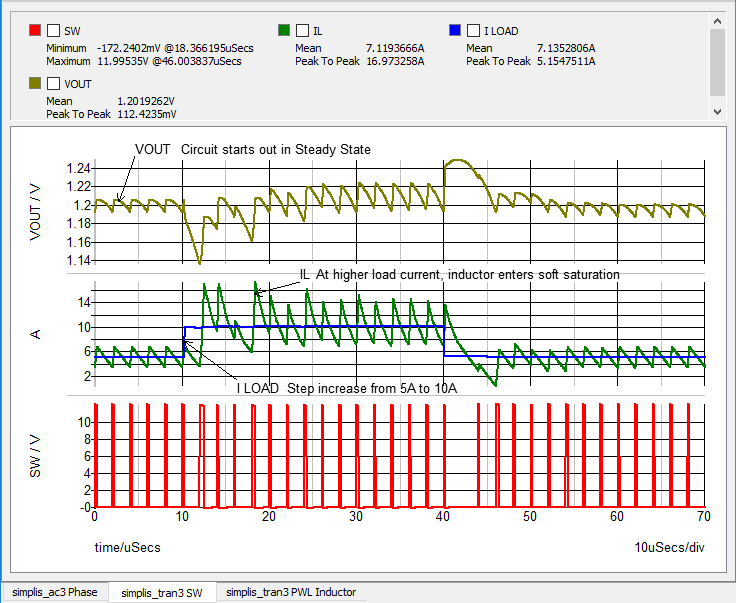1. Looking at the output voltage VOUT, note that the circuit starts out in Steady State.
2. The output load current increases by 5 A after 10 microseconds.
3. At higher load currents, the output inductor enters into soft saturation.
If we zoom in on the portion of the graph (by drawing a small box below the time axis) where the load current steps up from 5A to 10A,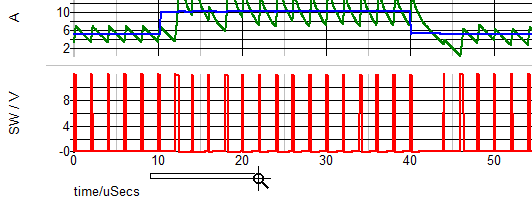we can clearly see (in the green curve labeled IL) the effects of soft saturation of the inductor L1.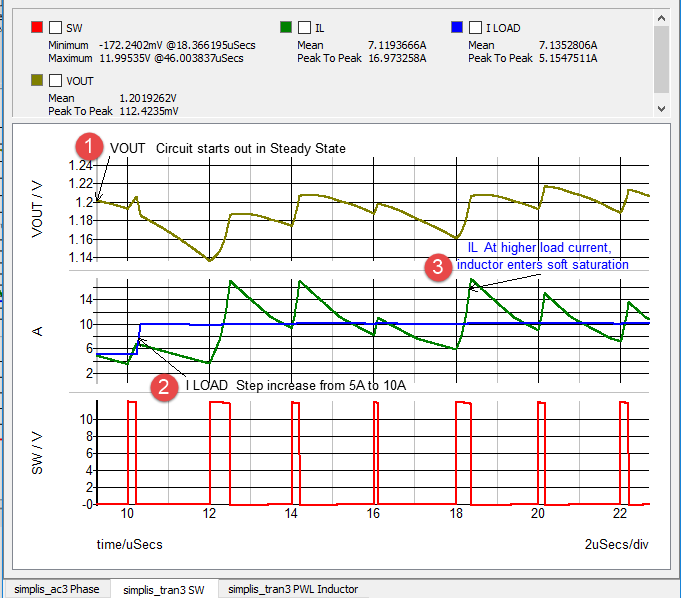• On the left tab we see the results of the SIMPLIS AC analysis displaying the Bode Plot of the closed loop response.
1. Loop Gain vs. Frequency.
2. Loop Phase vs. Frequency.
Note: The SIMPLIS AC analysis uses the full time-domain nonlinear switching model of the power supply. There is no need to derive an average model of the system!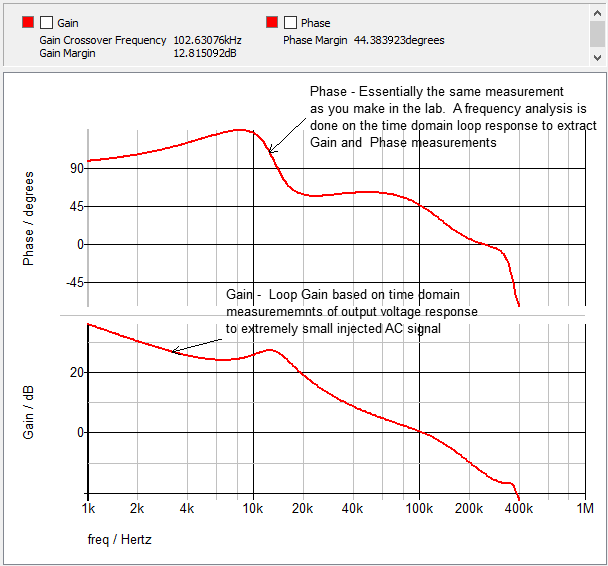• On the right tab we see a plot of the PWL inductor model characteristics in the flux linkages vs. current plane. this plot shows the piecewise linear magnetic model used to model soft saturation.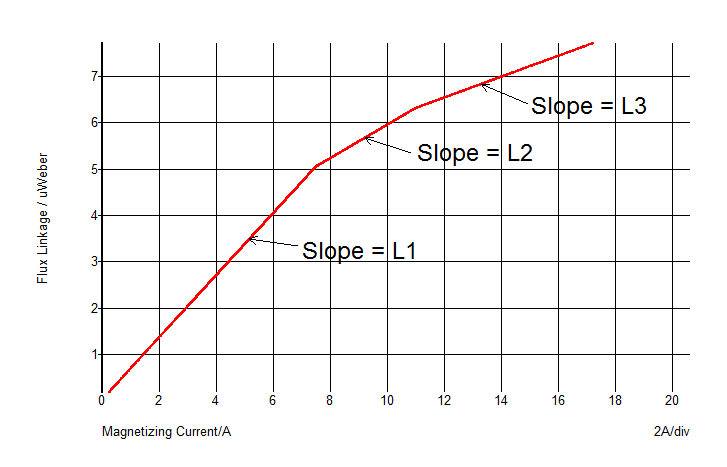These simulation results demonstrate the three SIMPLIS analysis modes: the Periodic Operating Point, AC and Transient analyses.

• The Periodic Operating Point (POP) analysis is a unique analysis mode that finds the steady-state operating waveforms of a periodic switching system.
• The SIMPLIS AC analysis finds the frequency response of a switching system without requiring a derivation of average models. This approach means that SIMPLIS does not need to make assumptions implicit in the derivation of averaged models, including that the switching frequency must be constant and that the magnitude of the ripple in the feedback signal path must be negligibly small. As a result SIMPLIS AC analysis is not limited to circuit topologies and control schemes where small-signal averaged models have already been derived.
• The SIMPLIS transient analysis is similar to that of SPICE but typically runs much faster and has far fewer mathematical "convergence" issues.

The example buck converter from the SIMPLIS Tutorial that we have been looking at is a good bit less complex than those we typically encounter in a production application. In each of the following schematics is an example application circuit of a real hardware reference design. Here you can observe the ability of SIMPLIS to address large complex production systems with accurate and very fast simulations.

Pick any one of the next three examples that you find interesting.

Each schematic is set up to first find the steady-state Periodic Operating Point of the system. It will then perform an AC analysis to find the Bode Plot of control loop of the full nonlinear time-domain switching system. Finally, it will perform a step-load transient. The approximate simulation times for each circuit are shown beside each link. The first number is the time to find the steady state Periodic Operating Point of the system, the second time is the time to perform the AC analysis, and the third time is that required for the time-domain step-load transient.

Schematic POP Simulation Time AC Simulation Time Transient Simulation Time
4-Phase Synchronous Buck 5s 3s 1s
6-Phase Synchronous Buck 14s 5s 3s
ZVS Full Bridge w/ Synchronous Rectified Output 22s 9s 2s

The speed, accuracy and lack of numerical convergence issues demonstrate how SIMPLIS makes it feasible to do detailed design verification before committing a design to build first hardware. The ability to find the steady-state periodic operating point of the system with great accuracy and repeatability in much less time than that required to run a long transient, opens up critical capabiliity to do design verification. This POP capability makes it possible to estimate switching losses accurately and repeatably. The POP analysis enables AC measurments without the need to derive average models of the system. This plus the lack of Spice-type convergence issues means that large complex systems can be designed and verified using SIMPLIS in a practical time frame for mission critical designs on tight schedules.

SIMetrix/SIMPLIS is the most widely used simulation tool for the design of closed-loop switching power supplies, primarily because SIMPLIS makes the analysis of large and complex systems practical for real-life design. This is accomplished based on SIMPLIS' ability to do the following:

• Quickly find the steady-state Periodic Operating Point of the system.
• Perform AC analyses on the full nonlinear time-domain switching circuit.
• Perform time-domain transient analyses 10 - 50 times faster than SPICE.
• Exhibit superior convergence behavior.

## Next Steps

1. Next, we encourage you to Read "SIMPLIS - The Rest of the Story" for an important description of how SIMPLIS works. This should only take a few minutes longer than it took you to go through this section.
2. After that, we suggest that you go through the SIMPLIS Tutorial to get an excellent hands-on introduction to creating schematics and running simulations in SIMPLIS.
3. After going through the SIMPLIS Tutorial you will be ready to make good use of a free SIMetrix/SIMPLIS Evaluation License. This Evaluation License will provide you with access to a time-limited license with the full capabilities of SIMetrix/SIMPLIS, without the circuit size limitations of SIMetrix/SIMPLIS Elements which you may download for free.